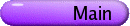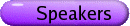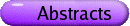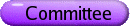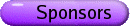Calabi-Yau Varieties and Mirror Symmetry Org: James Lewis (Alberta) and Noriko Yui (Queen's)[PDF] REZA AKHTAR, Miami University, Oxford, Ohio 45056, USA Chow-Kunneth and Lefschetz decompositions for quotients of abelian varieties [PDF] Let A be an abelian variety and G a finite group acting on A. The quotient variety A/G is not necessarily an abelian variety or even smooth. We discuss the motive of A/G, in particular how one can construct explicit Chow-Kunneth and Lefschetz decompositions for this motive in terms of the corresponding decompositions for the motive of A. This is joint work with Roy Joshua. MARIE JOSÉ BERTIN, University Paris 6, Institut de Mathématiques, 175 Rue du Chevaleret, 75013 Paris, France Mahler's measure and L-series of K3 hypersurfaces [PDF] The Mahler measure of a multivariate polynomial has been introduced by Mahler in 1962 to measure the size of factors of polynomials. Later in 1996, Deninger guessed a link between the Mahler measure of a certain polynomial defining an elliptic curve and the L-series of the curve. I plan to show how these facts extend to K3-hypersurfaces. I give several examples of singular K3-hypersurfaces for which the Mahler measure is expressed in terms of the L-series of the variety plus eventually a term related to the faces of the Newton polyhedron. VINCENT BOUCHARD, Perimeter Institute, 31 Caroline Street North, Waterloo, Ontario, N2L 2Y5, Canada On the landscape of standard-model bundles on non-simply connected Calabi-Yau threefolds [PDF] Let X be a smooth Calabi-Yau threefold of Schoen's type, i.e., a fiber product of two rational elliptic surfaces over P1. We classify all pairs (X,G), where G is a finite group acting freely on X, such that the quotient X/G is a non-simply connected torus-fibered Calabi-Yau threefold. We also systematize the construction of stable G-invariant vector bundles on X with structure group SU(4) or SU(5). This work is motivated by the search for vacua of heterotic string theory yielding realistic four-dimensional physics, and the study of the landscape of such heterotic vacua. This is joint work with Ron Donagi. XI CHEN, University of Alberta, Edmonton, AB, T6G 2G1 Vojta's 1+epsilon Conjecture and Bergman metric [PDF] I'll talk about a proof of Vojta's 1+epsilon conjecture. One interesting aspect of this proof is an application of Bergman metric. ADRIAN CLINGHER, University of Missouri in St. Louis On a special class of lattice polarized K3 surfaces [PDF] Using standard Hodge theory, one can associate to any K3 surface which is polarized by the rank 17 even lattice H+E8+E7 an abelian surface A(C) obtained as the jacobian of a genus-two curve. I will discuss a geometric method that leads to an explicit description of the correspondence. This is joint work with Charles Doran (University of Washington). CHUCK DORAN, University of Washington, Seattle, WA, USA On Stokes Matrices of Calabi-Yau Hypersurfaces [PDF] We consider Laplace transforms of the Picard-Fuchs differential equations of Calabi-Yau hypersurfaces and calculate their Stokes matrices. We also introduce two different types of Laplace transforms of Gel'fand-Kapranov-Zelevinski hypergeometric systems. This is joint work with Shinobu Hosono (University of Tokyo). YASUHIRO GOTO, Hokkaido University of Education, 1-2 Hachiman-cho, Hakodate, 040-8567 Japan Formal groups of Calabi-Yau threefolds in positive characteristic [PDF] Calabi-Yau varieties (over an algebraically closed field of positive characteristic) are associated with formal groups of dimension one and they are classified by the height. The height of the formal group of a K3 surface is bounded by 10 when it is finite. The existence of such a bound for Calabi-Yau threefolds is unknown. In this talk, we compute the height of formal groups of various Calabi-Yau threefolds arising from weighted Fermat/Delsarte threefolds and analyze its behavior. SHENGDA HU, Université de Montréal, Département de Math. et de Stat., CP 6128, succ. Centre-Ville, Montreal, QC, H3C 3J7, Canada T-duality with H-flux from generalized Kähler geometry [PDF] The construction of reduction by Poisson Lie group action in Poisson geometry can be extended to generalized complex geometry. A generalized Kähler manifold has two compatible generalized complex structures. We present a construction in generalized Kähler geometry via Poisson Lie reduction on either of the generalized complex structure. The two quotient manifolds thus obtained are possible candidates of a T-dual pair with H-flux. MATT KERR, University of Chicago (Math Dept.), 5734 S. University Ave Algebraic K-theory and local mirror symmetry [PDF] We discuss an aspect of recent joint work with C. Doran, relating our construction of families of cycles in higher K-theory to the mirror map in local mirror symmetry, and from there to asymptotics of Gromov-Witten invariants. NAM-HOON LEE, Korea Institute for Advanced Study Some attempt at constructing infinitely many families of Calabi-Yau manifolds [PDF] Some Calabi-Yau construction by smoothing normal crossings, that may possibly lead to construction of infinitely many families of Calabi-Yau manifolds, will be discussed. It depends on the existence of a specific type of threefolds and some K3 surfaces. As byproducts, we will also discuss some generalization of Enriques Calabi-Yau threefolds. JAMES LEWIS, University of Alberta The Abel-Jacobi Map for Higher Chow Groups, II [PDF] Let X be a projective algebraic manifold, and Y in X a normal crossing divisor. We describe the regulator map (on the level of complexes) from Bloch's higher Chow groups of X-Y into absolute Hodge cohomology, and give a description of the corresponding Abel-Jacobi map. This is based on joint work with Matt Kerr. BONG H. LIAN, Brandeis University and NUS Counting Fourier-Mukai Partners [PDF] I will discuss an explicit formula for counting Fourier-Mukai partners of any given algebraic K3 surface. This is joint work with Hosono, Oguiso and Yau. LING LONG, Iowa State University On the Coefficients of Noncongruence Modular Forms [PDF] By a theorem of Belyi, it is known that meromorphic functions on compact smooth orientable Riemann surfaces defined over the algebraic closure of Q are modular functions for finite index subgroups of the modular group. Predominately, most of these modular functions are for noncongruence subgroups. It was observed by Atkin and Swinnerton-Dyer that the Fourier coefficients having unbounded denominators is a clear distinction between noncongruence and congruence modular forms. However, it is unknown whether the coefficients of a genuine noncongruence modular form (with algebraic coefficients) will have unbounded denominators. In this talk, we will discuss the unbounded denominator property satisfied by the coefficients of several types of noncongruence modular forms, including some meromorphic functions parameterizing Calabi-Yau manifolds. STEVEN LU, Université du Québec à Montréal, Dépt. de Math., CP 8888 Succursale Centre-ville, Montréal, QC, H3C 3P8 On complex invariant metrics for some Calabi-Yau varieties [PDF] I will comment on some recent ideas of Claire Voisin on constructing invariant measures so as to show that they vanish on some Calabi-Yau varieties. The natural construction obtained is used to give the vanishing of a corresponding invariant metric for these varieties. JOHN MCKAY, Concordia University Towards an understanding of Monstrous Moonshine [PDF] We are working towards a better understanding of the geometry of the monster simple group, ultimately leading to a reduction in the number of sporadic finite simple groups. Some problems arising will be explained. MATTHIAS SCHUETT, Harvard University Classifying singular K3 surfaces [PDF] A complex K3 surface is called singular if it has Picard number 20. Singular K3 surfaces behave in many ways like elliptic curves with complex multiplication. For instance, they are defined over some number field. After reviewing some classical results, as of Shioda-Inose and Shafarevich, we will discuss the problem which singular K3 surfaces actually can be defined over Q. ANDREY TODOROV, University of California at Santa Cruz Regularized Determinants of CY Metrics, Borchards Products; Applications to K3 Surfaces [PDF] In this talk we will prove that there exists a holomorphic section of the relative dualizing sheaf over some finite cover of the moduli space of polarized CY manifolds whose L2 norm is equal to the regularized determinant of the CY metric of the Laplacian acting on (0,1) forms. We will show that in case of M-polarized K3 surfaces this section can be represented as Borcherds' product. Its logarithmic derivative counts nonsingular rational curves on K3 surface when M is an unimodular lattice. JOHANNES WALCHER, Institute for Advanced Study, Einstein Drive, Princeton, NJ 08540, USA Open Mirror Symmetry on the Quintic [PDF] The physics of string theory leads to interesting enumerative results for curves in Calabi-Yau manifolds. The most recent example concerns the number of holomorphic disks ending on the real Lagrangian in the quintic three-fold. This prediction has now been proven and can also be interpreted in the context of homological mirror symmetry. The open mirror theorem is joint work with R. Pandharipande and J. Solomon. SHING-TUNG YAU, Harvard University Complex manifolds with torsion [PDF] My talk will be on the construction of solution to a supersymmetric configuration for the compactification of Heterotic string model due to Strominger. It consists of constructing nonKahler manifolds with hermitian metrics coupled with Hermitian Yang Mills connections. JENG-DAW YU, Queen's University, Kingston, ON, Canada Local structure on non-supersingular Newton strata of K3 surfaces [PDF] Over finite characteristic, the moduli space of K3 surfaces has a natural stratification according to the heights of the associated formal Brauer groups. We show that the formal completion of a non-supersingular stratum at a point has an extension structure by a formal group. NORIKO YUI, Queen's University Motives, Mirror Symmetry and Modularity [PDF] We consider certain families of Calabi-Yau orbifolds and their mirror partners constructed from Fermat hypersurfaces in weighted projective spaces. We use Fermat motives to interpret the topological mirror symmetry phenonemon. These Calabi-Yau orbifolds are defined over Q, and we can discuss the modularity of the associated Galois representations. We address the modularity question at motivic level. We give some examples of modular Fermat motives. We then formulate a modularity conjecture about rank 4 Fermat motives that there exist Siegel modular forms on some congruence subgroups of Sp(4,Z).